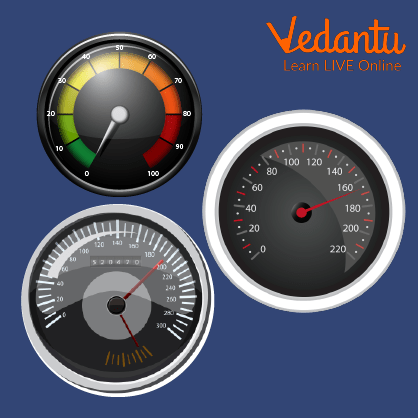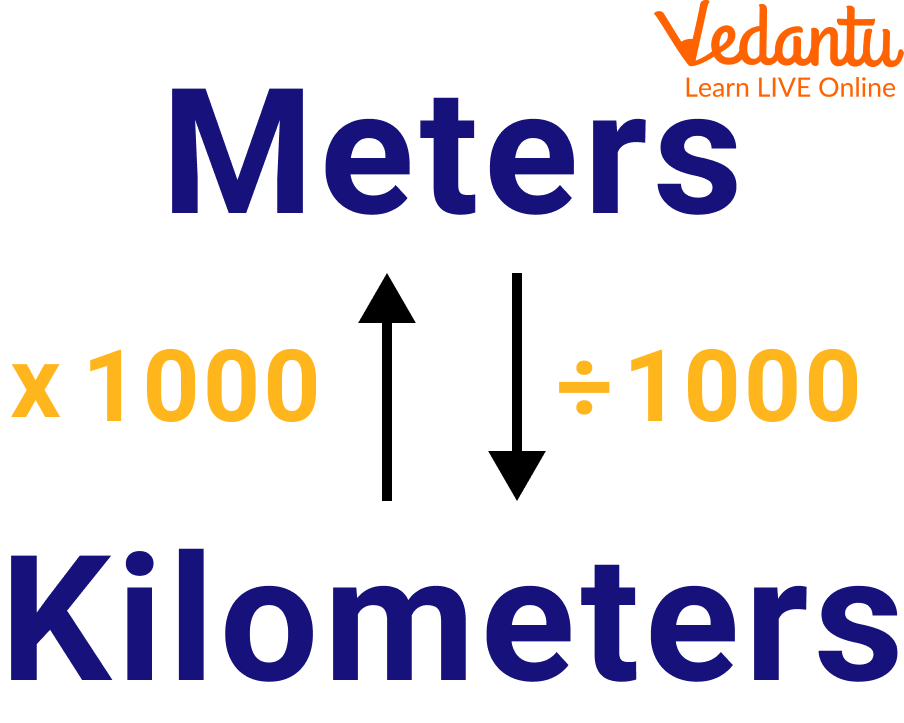Courses
Courses for Kids
Free study material
Free LIVE classes
More

# Learn How to Convert Kilometer Per Hour Into Meter Per SecondLIVE
Join Vedantu’s FREE Mastercalss

## Introduction to Units of Speed

Hello champs, you must have seen moving vehicles on the road, but, have you noticed different vehicles run at different speeds? Do you know how their speed is measured?

In this article, you will find all these answers and will be learning things that will help you to find accurate measurements of speed and time. The speed of the vehicle is measured in kilometers per hour. Conversion is important because suppose we are given a car which has a speed of 60 km/h and a bike with the speed of 220 m/s, so we need a uniform unit to compare them both.Police Charging Fine for Crossing the Speed Limit

## Converting Kilometer Per Hour to Meter Per Second

To convert a kilometer per hour measurement to a meter per second measurement, multiply the speed by the transformation ratio.

Since, one kilometer per hour = $\dfrac{\text{1 km}}{1\,\text{hr}}=1\times \dfrac{\text{1000 m}}{60\times 60\,\sec }$

$=\dfrac{1000}{3600}\text{m}\,\text{/}\,\text{s}$

$\text{=}\dfrac{5}{18}m/s$

So, we can say that to convert km/hr into m/sec, we multiply by $\dfrac{5}{18}$.

meters per second, you can use this simple equation to convert:

meters per second = $\mathrm{Kilometers per hour} \times \dfrac{5}{18}m/s$.

The speed in meters per second is equivalent to the kilometers per hour multiplied by $\dfrac{5}{18}$.

The meter per second is a SI unit of speed in the metric standard for measuring. Kilometers per hour can be abbreviated as km/h, and also some of the time abbreviated as kph. Also, 1 kilometer per hour can be written as 1 km/h or 1 kph.

## How to Convert Sec to hr?

We know 1 second is equal to $\dfrac{1}{3600}$ hour

Hence, the Seconds to Hours Conversion Formula is given as

Hours = Seconds ÷ 3,600

As discussed above, similarly, we can convert hr to sec by the following formula-

Seconds = Hours × 3,600.

## What is the Unit of Speed?

Meters per second is a SI unit of speed in the metric standard for measuring. It is the measurement of speed expressing the distance traveled in meters in a second.

Meters per second can be abbreviated as m/s, and are also abbreviated as m/sec. For example, 1 meter per second can also be written as 1 m/s or 1 m/sec.

Similarly, a kilometer per hour is also a unit of speed.

It can be abbreviated as km/h, and some of the time abbreviated as kph.

For example, 1 kilometer per hour can also be written as 1 km/h or 1 kph.

Kilometers per hour are a measurement of speed expressing the distance traveled in kilometers in an hour.Speedometer (Used to Measure the Speed of a Vehicle in km/hr)

## How to Convert km to m?Conversion

To convert a kilometer measurement to a meter measurement, multiply the length by the transformation ratio.

Since one kilometer is equivalent to 1,000 meters, you can use this basic formula to convert:

meters = kilometers × 1,000

The length in meters is equivalent to the kilometers multiplied by 1,000.

For example, this is the way to convert 8 kilometers to meters using the formula above.

8 km = (8 × 1,000) = 8,000 m

## Steps to Convert m Into Km

Step 1: For example, take 2,000 meters.

Step 2: Since one kilometer is equal to 1,000 meters, Divide it by 1,000.

Step 3: By doing so, 2,000 m = (2,000 ÷ 1,000) = 2 Km

## Solved Examples

Q 1. Convert 54 km/hr to m/sec

Ans: We have 54 km/hr

= $54\mathrm{km/hr} \times \dfrac{5}{18}m/s$

= 15 m/sec

Q 2. The speed of the bicycle is 36 km/hr, what is its speed in m/sec?

Ans: Speed of bicycle = 36 km/hr

We know that to convert the speed into m/sec, we multiply by $\dfrac{5}{18}$

Therefore, speed of bicycle = $36\mathrm{km/hr} \times \dfrac{5}{18}m/s$

= 10m/s

## Practice Questions

Q 1. Convert 36 km/s into m/s.

Ans: 10m/s

Q 2. Convert 54 km/s into m/s.

Ans: 15m/s

Q 3. Convert 10 km into m.

Ans: 10,000 m

Q 4. Convert 10,000 m into km.

Ans: 10 Km

Q 5. Convert 40,000 m into km.

Ans: 40 Km

## Summary

In this article, we discussed the kilometer per hour, which is a SI unit of speed. To convert a kilometer per hour measurement to a meter per second measurement, multiply the speed by the conversion ratio. We multiply km/hr by $\dfrac{5}{18}$ to get data in m/sec. Km per hr is an example of the speed we measure while running a vehicle. At the end of the article, we added some practice questions, so after going through the article, give it a try to the practice questions.

Last updated date: 24th Sep 2023
Total views: 98.1k
Views today: 0.98k

## FAQs on Learn How to Convert Kilometer Per Hour Into Meter Per Second

1. What is the purpose behind the unit conversion?

A unit change expresses the same property as a different unit of measurement. For example, time can be expressed in minutes rather than hours, while distance can be converted from miles to kilometers, meters, feet, or some other proportion of length. Frequently measurements are given in one set of units, like feet, yet are required in different units, like inches. A conversion factor is a numeric expression that enables feet to be changed to inches as an equivalent exchange.

2. What are the ways to calculate the speed of a vehicle?

The speed of a vehicle is calculated by dividing distance by time. The most well-known units of speed are meters each second (m/s), kilometers per hour (km/h), and miles per hour (mph). In a typical car journey, the speed of the vehicle will change. The distance divided by the time will calculate the vehicle's average speed. A few devices like a speedometer are fixed inside the vehicle to calculate the speed and speed gun to see while pointing at a vehicle.

3. What is a kilometer?

Kilometer is the unit of length measurement. It is equal to 1000 meters. It is denoted by the symbol km.